Microcontroller projects

Oscilloscope clock with internet time

last updated: 11/08/18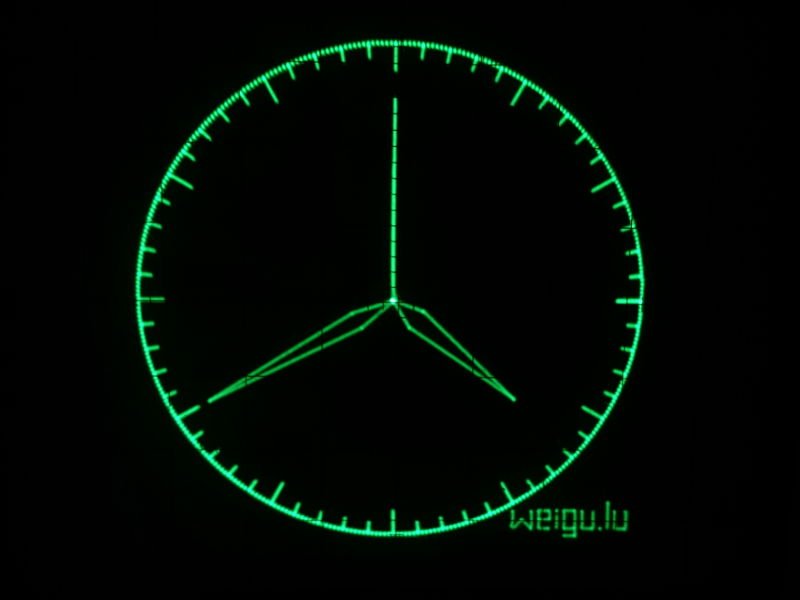Introduction

Old Oscilloscopes want to live forever. To do so, here is the oscilloscope clock. The code is written to get a clear image of a clock with no ghost lines. The hands are drawn beginning from the origin and back to the origin. Strokes are drawn during drawing the circle.

Teensy 3.6 with internal RTC is quick enough to use mathematical functions. With his two 12 Bit DAC, the picture is rock stable and sharp. The only hardware needed is an external battery for the RTC. The Teensy has no direct connection to the Internet and no DCF77, so the time may be getting imprecise over the year.

The new ESP32 has also two DAC with 8 Bit and the possibility to get the time over Internet, but it is not quick enough to use mathematical functions because of his operating system running in background (RTOS). It was even necessary to create tables for the circle and the hands and to reduce the points of the circle to 180 to get a stable non flickering image.

So the best solution is the Teensy with an ESP8266 to get NTP from Internet. The ESP8266 is sending once a day (4 AM) the time to the Teensy. If synchronising in the night was successful the text "NTP" is displayed until 18 hour.

Oscilloscope clock with Teensy 3.6

Teensy 3.6 has an internal RTC (and crystal) and 2 DAC with 12 Bit. So no other hardware is needed then the Teensy-Board to create a nice oscilloscope clock.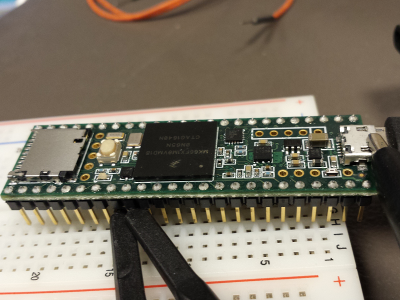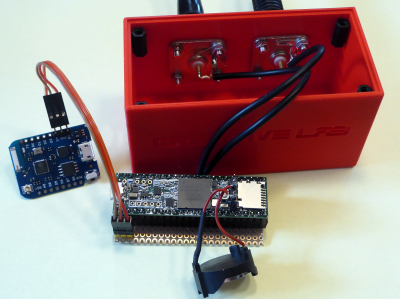All the magic is in the code. The Teensy is quick enough to calculate the needed points for the clock, so we can omit tables. The clock strokes are drawn from the circle line back and forth, so no ghost lines appear. Same thing for the second line and hands. The resolution can be changes from 8 Bit to 12 Bit. 12 Bit gets a real sharp, steady nice image of the clock.

The best solution is adding an ESP8266 to get NTP from Internet. The ESP8266 is sending once a day (4 AM) the time to the Teensy.

Her is the Arduino code with ESP8266 (D4, TXD) connected to pin1 (RXD1). The other code (for ESP8266 or Teensy without ESP) can be found under Downloads at the endof the page.

// oscilloscope clock with teensy 3.6
// weigu.lu v3 with NTP over serial
// 11/08/18

#include <TimeLib.h> //https://www.pjrc.com/teensy/td_libs_Time.html

const byte dac1 = A21;
const byte dac2 = A22;
const float pi = 3.14159265;
const byte res = 12;       // dac resolution in bit
int r = (pow(2,res)-2)/2;  // max circle radius
int rs = r*0.80;           // radius seconds
int rm = r*0.85;           // radius minutes
int rh = r*0.65;           // radius hours
int rst = r*0.95;          // radius strokes
int rstm = r*0.90;         // radius main strokes
int hands = 5;             // fraction where to split the hand
int handam = 12;           // angle (width) of hand (min)
int handah = 20;           // angle (width) of hand (hour)
byte NTP_flag = 0;

void setup() {
Serial1.begin(115200);
setSyncProvider(getTeensy3Time); // set the time library to use RTC
analogWriteResolution(res);
}

void loop() {
if (Serial1.available()) {
time_t t = processSyncMessage();
if (t != 0) {
Teensy3Clock.set(t); // set the RTC
setTime(t);
NTP_flag = 1;
}
}
circle();    // function to make a circle
sec_line(second());
min_line(minute());
hour_line(hour(),minute(),second());
display_weigu(r*15/10,r/32,r/64);
if (NTP_flag) {
display_NTP(r*18/10,r*19/10,r/64);
}
if (hour()>18) {
NTP_flag = 0;
}
}

time_t getTeensy3Time() {
return Teensy3Clock.get();
}

void point(int x,int y) {
analogWrite(dac1, y);
analogWrite(dac2, x);
}

void line (int x1,int y1,int x2,int y2) { // draw a line between 2 points
int d = sqrt(((x2-x1)*(x2-x1))+((y2-y1)*(y2-y1)));
int steps = d/4;
for(int i=0;i<steps;i++) {
point(x1+i*(x2-x1)/steps,y1+i*(y2-y1)/steps);
}
}

void dline (int x1,int y1,int x2,int y2) { // draw a line back and forth
int d = sqrt(((x2-x1)*(x2-x1))+((y2-y1)*(y2-y1)));
int steps = d/4;
for(int i=0;i<steps;i++) {
point(x1+i*(x2-x1)/steps,y1+i*(y2-y1)/steps);
}
for(int i=0;i<steps;i++) {  //draw line backwards to avoid ghosts
point(x2+i*(x1-x2)/steps,y2+i*(y1-y2)/steps);
}
}

void hand (int x1,int y1,int x2,int y2, int angle) { // draw a hand between 2 points
int d = sqrt(((x2-x1)*(x2-x1))+((y2-y1)*(y2-y1)));
int ds = d/hands;
int ao = round( atan2 (y2-y1,x2-x1)*180/pi );
int a1 = ao+angle;
int a2 = ao-angle;
int p1x = x1+ds*cos(a1*pi/180);
int p1y = y1+ds*sin(a1*pi/180);
int p2x = x1+ds*cos(a2*pi/180);
int p2y = y1+ds*sin(a2*pi/180);
line (x1,y1,p1x,p1y);
line (p1x,p1y,x2,y2);
line (x2,y2,p2x,p2y);
line (p2x,p2y,x1,y1);
}

void circle() { //function to draw a circle
for(int i=0;i<=360;i++) {
int circlex = r*cos(i*pi/180)+r;
int circley = r*sin(i*pi/180)+r;
point(circlex,circley);
if (i%6==0) {  // min lines
if (i%30==0) {  // 5 min lines
dline(circlex,circley,rstm*cos(i*pi/180)+r,rstm*sin(i*pi/180)+r);
}
else {
dline(circlex,circley,rst*cos(i*pi/180)+r,rst*sin(i*pi/180)+r);
}
}
}
}

void  sec_line(int second) {
int angle = ((60-second)*6+90)%360;
dline(r,r,rs*cos(angle*pi/180)+r,rs*sin(angle*pi/180)+r);
}

void  min_line(int minute) {
int angle = ((60-minute)*6+90)%360;
hand(r,r,rm*cos(angle*pi/180)+r,rm*sin(angle*pi/180)+r,handam);
}

void  hour_line(int hour, int minute, int second) {
if (hour>12) {
hour = hour-12;
}
int hours = hour*3600 + minute*60 + second;
int angle = ((43200-hours)/120+90)%360;
hand(r,r,rh*cos(angle*pi/180)+r,rh*sin(angle*pi/180)+r,handah);
}

void display_weigu(int x1, int y1, int s) {
int h = 5*s; // height of chars
int w = 5*s; // width of chars
int d = 2*s; // distance between chars
line(x1,y1,x1,y1+h);
line(x1,y1,x1+w/2,y1+h/2);
line(x1+w/2,y1+h/2,x1+w,y1);
line(x1+w,y1,x1+w,y1+h);
x1 = x1+w+d;
w = w*3/5;
line(x1,y1,x1,y1+h);
line(x1,y1+h,x1+w,y1+h);
line(x1+w,y1+h,x1+w,y1+h/2);
line(x1+w,y1+h/2,x1,y1+h/2);
line(x1,y1,x1+w,y1);
x1 = x1+w+d;
line(x1,y1,x1,y1+h*3/4);
point(x1,y1+h);
point(x1+1,y1+h);
point(x1+1,y1+1+h);
point(x1,y1+1+h);
x1 = x1+d;
line(x1+w,y1,x1,y1);
line(x1,y1,x1,y1+h);
line(x1,y1+h,x1+w,y1+h);
line(x1+w,y1+h,x1+w,y1);
line(x1+w,y1,x1+w,y1-h/2);
line(x1+w,y1-h/2,x1,y1-h/2);
x1 = x1+w+d;
line(x1,y1,x1,y1+h);
line(x1,y1,x1+w,y1);
line(x1+w,y1,x1+w,y1+h);
x1 = x1+w+d;
point(x1,y1);
point(x1+1,y1);
point(x1+1,y1+1);
point(x1,y1+1);
x1 = x1+d;
line(x1,y1,x1,y1+1.5*h);
x1 = x1+d;
line(x1,y1,x1,y1+h);
line(x1,y1,x1+w,y1);
line(x1+w,y1,x1+w,y1+h);
}

void display_NTP(int x1, int y1, int s) {
int h = 5*s; // height of chars
int w = 3*s; // width of chars
int d = 2*s; // distance between chars
line(x1,y1,x1,y1+h);
line(x1,y1+h,x1+w,y1);
line(x1+w,y1+h,x1+w,y1);
x1 = x1+w+d;
//w = w*3/5;
line(x1+w/2,y1,x1+w/2,y1+h);
line(x1+w/2,y1+h,x1,y1+h);
line(x1,y1+h,x1+w,y1+h);
line(x1+w,y1+h,x1+w/2,y1+h);
line(x1+w/2,y1+h,x1+w/2,y1);
x1 = x1+w+d;
line(x1,y1,x1,y1+h);
line(x1,y1+h,x1+w,y1+h);
line(x1+w,y1+h,x1+w,y1+h/2);
line(x1+w,y1+h/2,x1,y1+h/2);
line(x1,y1+h/2,x1,y1);
}

/*  code to process time sync messages from the serial port  pjrc.com */
#define TIME_HEADER  "T"   // Header tag for serial time sync message

unsigned long processSyncMessage() {
unsigned long pctime = 0L;
const unsigned long DEFAULT_TIME = 1533987381; // 11/8/18
pctime = Serial1.parseInt();
return pctime;
if( pctime < DEFAULT_TIME) { // check the value is a valid time (greater than Jan 1 2013)
pctime = 0L; // return 0 to indicate that the time is not valid
}
}
return pctime;
}

Oscilloscope clock with ESP32

The ESP32 (Lolin32) don't need additional components.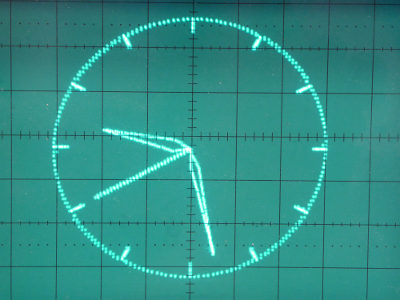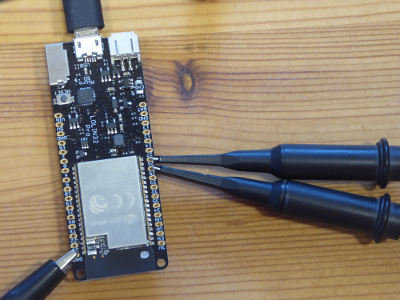// oscilloscope clock with wemos lolin32 (esp32)
// weigu.lu
// (reduce upload speed if errors occur)
// for tables see libreoffice calc sheet

#include <WiFi.h>
#include "time.h"
#include <driver/dac.h>

const byte dac1 = 25;
const byte dac2 = 26;

const char* ssid       = "myssid";
const char* password   = "mypass";

const char* ntpServer = "pool.ntp.org";
const long  gmtOffset_sec = 3600;
const int   daylightOffset_sec = 3600;
struct tm ti;

// sine and cosine table with 360 points
unsigned int circlex[]={
255,255,255,255,255,255,254,254,
...
};

unsigned int circley[]={
128,130,132,134,136,139,141,143,
...
};

// 5 min strokes
unsigned int min5linesxy[]={
243,128,227,185,185,227,128,243,
...
};

// seconds hand r = 102 (80%)
unsigned int secHandxy[]={
128,230,117,229,106,227,96,225,
...
};

// minute hand r = 108 (85%) fraction =  5 angle = 12
unsigned int minHand[]={
128,236,123,149,132,149,
...
};

// hour hand r = 83 (65%) fraction =  5 angle = 20
unsigned int hourHand[]={
128,211,122,143,133,143,
...
};

void setup() {
Serial.begin(115200);
//connect to WiFi
Serial.printf("Connecting to %s ", ssid);
WiFi.enableSTA(true);
while (WiFi.status() != WL_CONNECTED) {
delay(500);
Serial.print(".");
}
Serial.println(" CONNECTED");
//init and get the time
configTime(gmtOffset_sec, daylightOffset_sec, ntpServer);
getLocalTime(&ti);
WiFi.disconnect(true); //disconnect WiFi as it's no longer needed
WiFi.mode(WIFI_OFF);
}

void loop() {
getLocalTime(&ti);
for(int i=0;i<5;i++) {
circle();    // function to make a circle
sec_hand(ti.tm_sec);
min_hand(ti.tm_min);
hour_hand(ti.tm_hour,ti.tm_min);
}
// Reset time at 4 in the morning
if ((ti.tm_hour == 4) and (ti.tm_min == 0) and (ti.tm_sec == 0)) {
ESP.restart();
}
}

void point(int x,int y) {
dac_out_voltage(DAC_CHANNEL_1, y);
dac_out_voltage(DAC_CHANNEL_2, x);
}

void line (int x1,int y1,int x2,int y2, int steps) { // draw a line between 2 points
for(int i=0;i<steps;i++) {
point(x1+i*(x2-x1)/steps,y1+i*(y2-y1)/steps);
}
}

void dline (int x1,int y1,int x2,int y2,int steps) { // draw a line back and forth
for(int i=0;i<steps;i++) {
point(x1+i*(x2-x1)/steps,y1+i*(y2-y1)/steps);
}
for(int i=0;i<steps;i++) {  //draw line backwards to avoid ghosts
point(x2+i*(x1-x2)/steps,y2+i*(y1-y2)/steps);
}
}

void circle() { //function to draw a circle
for(int i=0;i<360;i=i+2) {
point(circlex[i],circley[i]);
if (i%30==0) {  // 5 min lines
dline(circlex[i],circley[i],min5linesxy[i/30*2],min5linesxy[i/30*2+1],5);
}
}
}

void  sec_hand(int second) {
int i = (60-second);
if (i == 60) i = 0;
i = i*2;
dline(127,127,secHandxy[i],secHandxy[i+1],30);
}

void  min_hand(int minute) {
int i = (60-minute);
if (i == 60) i = 0;
i = i*6;
line (127,127,minHand[i+2],minHand[i+3],10);
line (minHand[i+2],minHand[i+3],minHand[i],minHand[i+1],40);
line (minHand[i],minHand[i+1],minHand[i+4],minHand[i+5],40);
line (minHand[i+4],minHand[i+5],127,127,10);
}

void  hour_hand(int hour, int minute) {
if (hour>12) hour = hour-12;
int hours = (hour*5 + (minute/12));
int i = (60-hours);
if (i == 60) i = 0;
i = i*6;
line (127,127,hourHand[i+2],hourHand[i+3],7);
line (hourHand[i+2],hourHand[i+3],hourHand[i],hourHand[i+1],30);
line (hourHand[i],hourHand[i+1],hourHand[i+4],hourHand[i+5],30);
line (hourHand[i+4],hourHand[i+5],127,127,7);
}

3D printed housing

Find the freecad file and the stl files under Downloads. I used 4 hole flange mount BNCs from my stock with 19 mm distance between the holes. Perhaps you have to adjust the distance in the freecad file.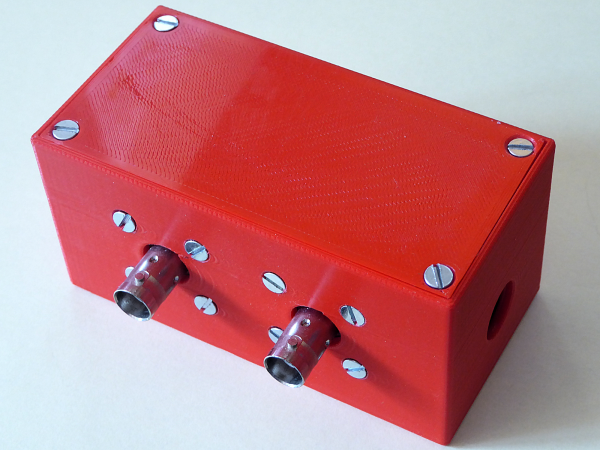The little piece of breadboard that holds the teensy is glued with hot glue to the bottom of the case.